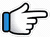# Tera Hua – Keyboard Notes – Dheere Dheere Se## Tera Hua – Dheere Dheere Se Tera Hua Keyboard Notes

For Tera Hua Notes as Western or Indian Notations, Click –

Western – CDE FGAB

Indian – srg mpdn

Hindi – सारेग मपधनि

## Keyboard Notes for Tera Hua – Indian Notation (srg mpdn)

CDE FGAB

सारेग मपधनि

Scale for Dheere Dheere Se Tera Hua is D Major.

Notes for D major scale are –

r, g, M, p, d, n, and R

So, the notes used for Tera Hua song are –

g, M, p, d, n,
R*, r*, g*, M* and d*

### Tips to play the song Tera Hua

Why this song is in easy to play song list –

In most of the lines of the song,
these are the only two lines of notes –

R*r* M*g*M*~ g*  r*R*n~  R*~n
Or
Mp d~ nr* R* n dM* dd~ pMg

So, by practising these two lines,
you can easily play the song.

Starting Music

Starting music notes are given
after the full song notes.

### Part 1

Tere qareeb aa raha hoon
Khud se main door jaa raha hoon
Yeh bewajah toh nahi hai
Tu jo mila~~

R*r*   M*g*M*~
Tere   qareeb

g*    r*R*n~  R*~n
aa   raha       hoon

R*       r*   M*      g*M*~
Khud   se  main   door

g*    r*R*n~   R*~ n
jaa   raha       hoon

R*     r* M* g*M*~
Yeh   bewajah

g*    r*R*n~  R*~n
toh   nahi      hai

d    M~   dd~ p~ M g
Tu   jo     mila~~

### Part 2

Dheere dheere se tera hua
Haule haule se tera hua
Rafta rafta tera hua
Tere bin main hoon be-nishaan

Mp         d~  nr* R* n
Dheere   dhee_re_    se

dM*   dd~ pMg
tera    hua~~~

Mp       d~  nr* R* n
Haule   hau_le_ se

dM*   dd~
tera    hua

Mpd~    nr*R*
Rafta    rafta

ndM*   dd~ pMg
tera     hua

R*r* n       M*d*  r*
Tere  bin   main  hoon

n~  r*r*~~
be-nishaan
Or
R*~ r*r*~~

### Repeat Part 2

Mp      d~  nr* R*n  dM* dd~ pMg
Dheere dhee_re_se     tera   hua~~~

Mp    d~  nr* R* n  dM*  dd~
Haule  hau_le_ se       tera  hua

Mpd~  nr*R*  ndM*  dd~ pMg
Rafta     rafta       tera      hua

R*r* n   M*d*  r*     n~  r*r*~~
Tere   bin  main   hoon  be-nishaan

### Part 3

Samjho zara, samjho ishaara
Tera hoon main saara ka saara

R*r*        M*g*M~
Samjho   zara,

M*g*r*    R*R*r*~ R* n
samjho   ishaara

R*r*    M*      g*M~
Tera   hoon   main

M*g*r*   R*  R*r*~
saara    ka   saara

### Part 4

Jaise mujhe tumse hua hai
Yeh pyar naa hoga dobara

R*r*    M*g*M~
Jaise  mujhe

M*g*r*  R*R*  r*~ R* n
tumse   hua     hai

R*    r*M*   g*M~
Yeh  pyar   naa

M*g*r*   R*R*r*~
hoga      dobara

### Part 5

Dil mein teri jo jagah hai
Uski koi toh wajah hai

Yeh bewajah toh nahi hai
Tu jo mila…

R*   r*       M*g*M~
Dil   mein   teri

g*    r*R*n~  R*~n
jo     jagah     hai

R* r*   M*g*M*~
Uski     koi

g*    r*R*n~   R*~ n
toh    wajah    hai

R*     r* M* g*M*~
Yeh   bewajah

g*    r*R*n~  R*~n
toh   nahi      hai

d    M~  dd p~ M g
Tu   jo    mila~~

### Repeat Part 2

Mp d~ nr* R*n dM* dd~ pMg
Dheere dhee_re_se tera hua~~~

Mp d~ nr* R* n dM* dd~
Haule hau_le_ se tera hua

Mpd~ nr*R* ndM* dd~ pMg
Rafta rafta tera hua

R*r* n M*d* r* n~ r*r*~~
Tere bin main hoon be-nishaan

### Starting Music

M*~ d*~~  M*~ d*~~
M*~ d*~~~

g*~ d*~~   g*~ d*~~
g*~ d*~~~

r*~ d*~~  r*~ d*~~
r*~ d*~~~

n d* R* d*

M*~ d*~~  M*~ d*~~  M*~ d*~~~
g*~ d*~~   g*~ d*~~   g*~ d*~~~
r*~ d*~~  r*~ d*~~  r*~ d*~~~
n d* R* d*

So, now the full keyboard notes for Dheere Dheere Se Tera Hua are –

## Keyboard Notes For Tera Hua

Starting Music

M*~ d*~~  M*~ d*~~  M*~ d*~~~  |  g*~ d*~~  g*~ d*~~  g*~ d*~~~
r*~ d*~~  r*~ d*~~  r*~ d*~~~  |  n d* R* d*

Repeat

M*~ d*~~  M*~ d*~~  M*~ d*~~~  |  g*~ d*~~  g*~ d*~~  g*~ d*~~~  |  r*~ d*~~  r*~ d*~~  r*~ d*~~~  |  n d* R* d*

Part 1

R*r*  M*g*M*~  g*  r*R*n~  R*~n  |  R*  r*  M*  g*M*~  g*  r*R*n~  R*~ n
Tere qareeb aa raha hoon  |  Khud se main door jaa raha hoon

R*  r* M* g*M*~  g*  r*R*n~  R*~n  |  d  M~  dd~ p~ M g
Yeh bewajah toh nahi hai  |  Tu jo mila~~

Part 2

Mp  d~  nr* R* n  dM*  dd~ pMg  |  Mp  d~  nr* R* n  dM*  dd~
Dheere dheere se tera hua  |  Haule haule se tera hua

Mpd~  nr*R*  ndM*  dd~ pMg  |  R*r* n  M*d*  r*  n~  r*r*~~
Rafta rafta tera hua  |  Tere bin main hoon be-nishaan

Repeat Part 2

Mp  d~  nr* R* n  dM*  dd~ pMg  |  Mp  d~  nr* R* n  dM*  dd~  |  Mpd~  nr*R*  ndM*  dd~ pMg  |  R*r* n  M*d*  r*  n~  r*r*~~
Dheere dheere se tera hua  |  Haule haule se tera hua  |  Rafta rafta tera hua  |  Tere bin main hoon be-nishaan

Part 3

R*r*  M*g*M~  M*g*r*  R*R*r*~ R* n  |  R*r*  M*  g*M~  M*g*r*  R*  R*r*~
Samjho zara, samjho ishaara  |  Tera hoon main saara ka saara

Part 4

R*r*  M*g*M~  M*g*r*  R*R*  r*~ R* n  |  R*  r*M*  g*M~  M*g*r*  R*R*r*~
Jaise mujhe tumse hua hai  |  Yeh pyar naa hoga dobara

Part 5

R*  r*  M*g*M~  g*  r*R*n~  R*~n  |  R* r*  M*g*M*~  g*  r*R*n~ R*~ n
Dil mein teri jo jagah hai  |  Uski koi toh wajah hai

R*  r* M* g*M*~  g*  r*R*n~  R*~n  |  d  M~  dd p~ M g
Yeh bewajah toh nahi hai  |  Tu jo mila…

Repeat Part 2

Mp  d~  nr* R* n  dM*  dd~ pMg  |  Mp  d~  nr* R* n  dM*  dd~  |  Mpd~  nr*R*  ndM*  dd~ pMg  |  R*r* n  M*d*  r*  n~  r*r*~~
Dheere dheere se tera hua  |  Haule haule se tera hua  |  Rafta rafta tera hua  |  Tere bin main hoon be-nishaan

Song List

Bhajan List

New Song List